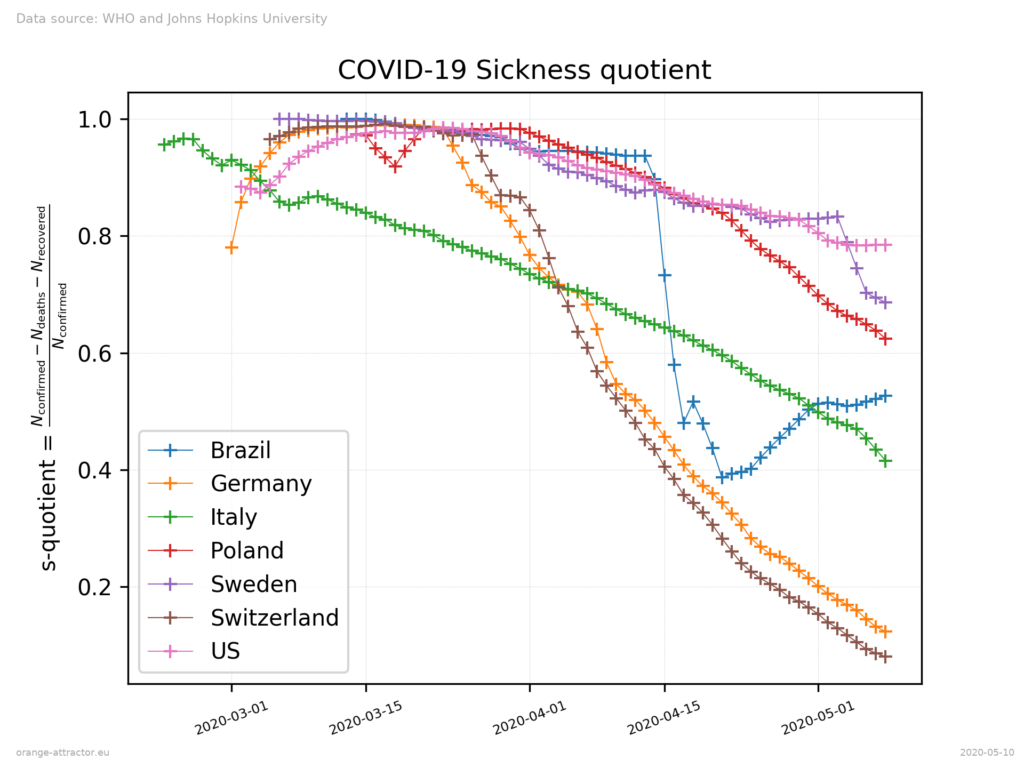# COVID-19 Sickness Quotient, can we predict the end of pandemic?

We present the behavior of COVID-19 “sickness quotient” for the selected countries.
As in the previous article, we use data published by  and provided by  for the following countries: Brazil, Germany, Italy, US, Poland, Sweden, Switzerland.
Sickness quotient is defined as follows:

$$S = \frac{N_{\mathrm{confirmed}} – N_{\mathrm{deaths}} – N_{\mathrm{recovered}}}{N_{\mathrm{confirmed}}},$$
Where $$N_{\mathrm{confirmed}}$$ is a total number of confirmed infections since the beginning of the pandemic, $$N_{\mathrm{deaths}}$$ is a number of deaths caused by the virus and $$N_{\mathrm{recovered}}$$ is a number of infected people who have been recovered. A simple moving average with window size 3 has been used in order to smooth the curve. We keep the convention used in many other publications – the plot starts at the date when the number of infected people is at least 100 for each country.Given s-quotient has two edge cases: $$S=1$$ means all infected individuals are still sick and no one died, $$S=0$$ means that all infected individuals are recovered or died. In the ideal model $$S$$ should go towards zero as the time goes. However we suspect that it might remain above the zero in the real population in some kind of stationary state.

As can be seen in the chart, there are several types of behavior. Germany and Switzerland go a similar path and their situation looks the best among the other presented countries, when only s-quotient change dynamics is taken into consideration. For Italy s-quotient drop is roughly constant since the beginning. For Poland it goes down steeper and should cross the Italy line assuming that this trend will continue. The US and Sweden seem to go both the same way. For Brazil… it looks that this s-quotient jumping is rather related to some data reporting problem than a real phenomena.

It’s tempting to try to predict the possible end of pandemic by fitting the straight line to the observed values for particular countries. So we did for the Italy case, resulting in cutting the zero line in about the second half of June (23 Jun). This estimate however, should be treated rather as the lower limit than the real target. As the China case shows – the s-quotient drop, changes its type over time and tends to be rather asymptotic. What is more, the behaviour of the trend depends on many different factors, some of them may or may not appear in the future. It would be rather surprising if such a simple model could predict rather complex phenomena.

Summary
Introduced sickness quotient allows monitoring of some basic aspects of the dynamics of the number of currently sick individuals changes. In spite of its linear nature in some ranges, predicting the end of pandemic for particular countries would require a much complex model.

References:
 World Health Organization
 John Hopkins University; Github repository.